## Identify Low-Order Transfer Functions (Process Models) Using System Identification App

### Introduction

#### Objectives

Estimate and validate simple, continuous-time transfer functions from single-input/single-output (SISO) data to find the one that best describes the system dynamics.

After completing this tutorial, you will be able to accomplish the following tasks using the System Identification app :

• Import data objects from the MATLAB® workspace into the app.

• Plot and process the data.

• Estimate and validate low-order, continuous-time models from the data.

• Export models to the MATLAB workspace.

• Simulate the model using Simulink® software.

Note

This tutorial uses time-domain data to demonstrate how you can estimate linear models. The same workflow applies to fitting frequency-domain data.

#### Data Description

This tutorial uses the data file `proc_data.mat`, which contains 200 samples of simulated single-input/single-output (SISO) time-domain data. The input is a random binary signal that oscillates between -1 and 1. White noise (corresponding to a load disturbance) is added to the input with a standard deviation of 0.2, which results in a signal-to-noise ratio of about 20 dB. This data is simulated using a second-order system with underdamped modes (complex poles) and a peak response at 1 rad/s:

`$G\left(s\right)=\frac{1}{1+0.2s+{s}^{2}}{e}^{-2s}$`

The sample time of the simulation is 1 second.

### What Is a Continuous-Time Process Model?

Continuous-time process models are low-order transfer functions that describe the system dynamics using static gain, a time delay before the system output responds to the input, and characteristic time constants associated with poles and zeros. Such models are popular in the industry and are often used for tuning PID controllers, for example. Process model parameters have physical significance.

You can specify different process model structures by varying the number of poles, adding an integrator, or including a time delay or a zero. The highest process model order you can specify in this toolbox is three, and the poles can be real or complex (underdamped modes).

In general, a linear system is characterized by a transfer function G, which is an operator that takes the input u to the output y:

`$y=Gu$`

For a continuous-time system, G relates the Laplace transforms of the input U(s) and the output Y(s), as follows:

`$Y\left(s\right)=G\left(s\right)U\left(s\right)$`

In this tutorial, you estimate G using different process-model structures.

For example, the following model structure is a first-order, continuous-time model, where K is the static gain, Tp1 is a time constant, and Td is the input-to-output delay:

`$G\left(s\right)=\frac{K}{1+s{T}_{p1}}{e}^{-s{T}_{d}}$`

### Preparing Data for System Identification

Load the data in `proc_data.mat` by typing the following command in the MATLAB Command Window:

```load proc_data ```

This command loads the data into the MATLAB workspace as the data object `z`. For more information about `iddata` objects, see the corresponding reference page.

#### Opening the System Identification App

To open the System Identification app , type the following command at the MATLAB Command Window:

`systemIdentification`

The default session name, `Untitled`, appears in the title bar.#### Importing Data Objects into the System Identification App

You can import data object into the app from the MATLAB workspace.

You must have already loaded the sample data into MATLAB, as described in Loading Data into the MATLAB Workspace, and opened the app, as described in Opening the System Identification App.

If you have not performed these steps, click here to complete them.

To import a data object into the System Identification app :

1. Select Import data > Data object.

This action opens the Import Data dialog box.2. In the Import Data dialog box, specify the following options:

• Object — Enter `z` as the name of the MATLAB variable that is the time-domain data object. Press Enter.

• Data name — Use the default name `z`, which is the same as the name of the data object you are importing. This name labels the data in the System Identification app after the import operation is completed.

• Starting time — Enter `0` as the starting time. This value designates the starting value of the time axis on time plots.

• Sample time — Enter `1` as the time between successive samples in seconds. This value represents the actual sample time in the experiment.

The Import Data dialog box now resembles the following figure.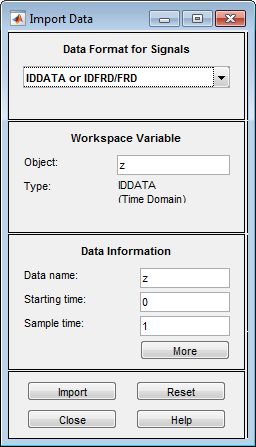3. Click to add the data to the System Identification app. The app adds an icon to represent the data.4. Click to close the Import Data dialog box.

#### Plotting and Processing Data

In this portion of the tutorial, you evaluate the data and process it for system identification. You learn how to:

• Plot the data.

• Remove offsets by subtracting the mean values of the input and the output.

• Split the data into two parts. You use one part of the data for model estimation, and the other part of the data for model validation.

The reason you subtract the mean values from each signal is because, typically, you build linear models that describe the responses for deviations from a physical equilibrium. With steady-state data, it is reasonable to assume that the mean levels of the signals correspond to such an equilibrium. Thus, you can seek models around zero without modeling the absolute equilibrium levels in physical units.

You must have already imported data into the System Identification app, as described in Importing Data Objects into the System Identification App.

If you have not performed this step, click here to complete it.

To plot and process the data:

1. Select the Time plot check box to open the Time Plot window.The bottom axes show the input data—a random binary sequence, and the top axes show the output data.

The next two steps demonstrate how to modify the axis limits in the plot.

2. To modify the vertical-axis limits for the input data, select Options > Set axes limits in the Time Plot figure window.

3. In the Limits for Time Plot dialog box, set the new vertical axis limit of the input data channel u1 to [-1.5 1.5]. Click and .Note

The other two fields in the Limits for Time Plot dialog box, Time and y1, let you set the axis limits for the time axis and the output channel axis, respectively. You can also specify each axis to be logarithmic or linear by selecting the corresponding option.

The following figure shows the updated time plot.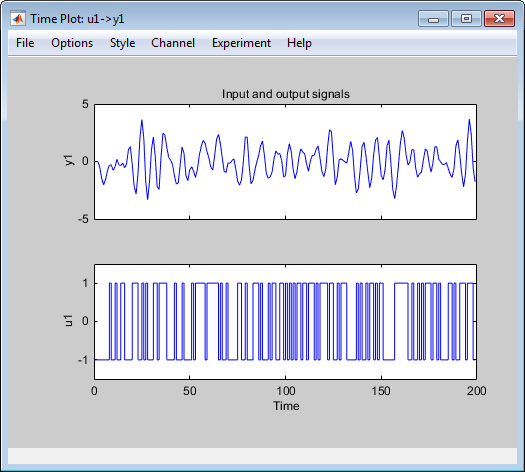4. In the System Identification app , select <--Preprocess > Quick start to perform the following four actions:

• Subtract the mean value from each channel.

• Split the data into two parts.

• Specify the first part of the data as estimation data (or Working Data).

• Specify the second part of the data as Validation Data.

Learn More.  For information about supported data processing operations, such as resampling and filtering the data, see Preprocess Data.

### Estimating a Second-Order Transfer Function (Process Model) with Complex Poles

#### Estimating a Second-Order Transfer Function Using Default Settings

In this portion of the tutorial, you estimate models with this structure:

`$G\left(s\right)=\frac{K}{\left(1+2\xi {T}_{w}s+{T}_{w}{}^{2}{s}^{2}\right)}{e}^{-{T}_{d}s}$`

You must have already processed the data for estimation, as described in Plotting and Processing Data.

If you have not performed this step, click here to complete it.

To identify a second-order transfer function:

1. In the System Identification app, select Estimate > Process models to open the Process Models dialog box.2. In the Model Transfer Function area of the Process Models dialog box, specify the following options:

• Under Poles, select `2` and `Underdamped`.

This selection updates the Model Transfer Function to a second-order model structure that can contain complex poles.

• Make sure that the Zero and Integrator check boxes are cleared to exclude a zero and an integrator (self-regulating ) from the model.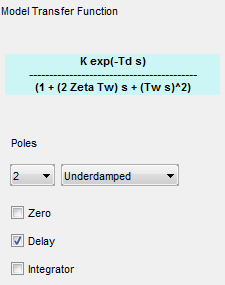3. The Parameter area of the Process Models dialog box now shows four active parameters: `K`, `Tw`, `Zeta`, and `Td`. In the Initial Guess area, keep the default `Auto-selected` option to calculate the initial parameter values during the estimation. The Initial Guess column in the Parameter table displays `Auto`.

4. Keep the default Bounds values, which specify the minimum and maximum values of each parameter.

Tip

If you know the range of possible values for a parameter, you can type these values into the corresponding Bounds fields to help the estimation algorithm. Press the Enter key after you specify the values.

5. Keep the default settings for the estimation algorithm:

• Disturbance Model`None` means that the algorithm does not estimate the noise model. This option also sets the Focus to `Simulation`.

• Focus`Simulation` means that the estimation algorithm does not use the noise model to weigh the relative importance of how closely to fit the data in various frequency ranges. Instead, the algorithm uses the input spectrum in a particular frequency range to weigh the relative importance of the fit in that frequency range.

Tip

The `Simulation` setting is optimized for identifying models that you plan to use for output simulation. If you plan to use your model for output prediction or control applications, or to improve parameter estimates using a noise model, select `Prediction`.

• Initial condition`Auto` means that the algorithm analyzes the data and chooses the optimum method for handling the initial state of the system. If you get poor results, you might try setting a specific method for handling initial states, rather than choosing it automatically.

• Covariance`Estimate` means that the algorithm computes parameter uncertainties that display as model confidence regions on plots.

The app assigns a name to the model, shown in the Name field (located at the bottom of the dialog box). By default, the name is the acronym `P2DU`, which indicates two poles (`P2`), a delay (`D`), and underdamped modes (`U`).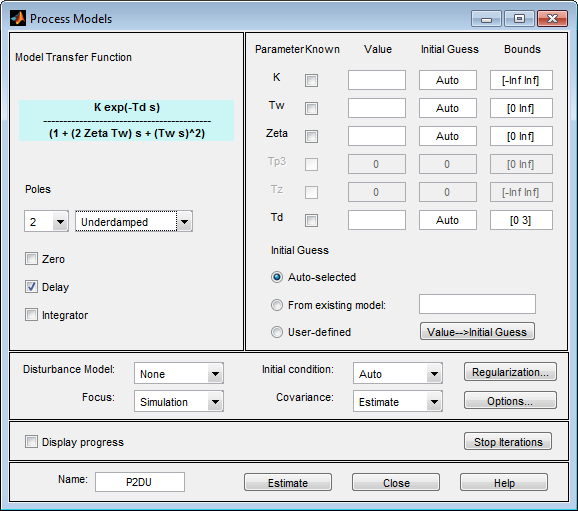6. Click to add the model `P2DU` to the System Identification app.

#### Tips for Specifying Known Parameters

If you know a parameter value exactly, you can type this value in the Value column of the Process Models dialog box. Select the corresponding Known check box after you specify the value.

If you know the approximate value of a parameter, you can help the estimation algorithm by entering an initial value in the Initial Guess column. In this case, keep the Known check box cleared to allow the estimation to fine-tune this initial guess.

For example, to fix the time-delay value `Td` at `2`s, type this value into Value field of the Parameter table in the Process Models dialog box. Then select the corresponding Known check box.#### Validating the Model

You can analyze the following plots to evaluate the quality of the model:

• Comparison of the model output and the measured output on a time plot

• Autocorrelation of the output residuals, and cross-correlation of the input and the output residuals

You must have already estimated the model, as described in Estimating a Second-Order Transfer Function Using Default Settings.

If you have not performed this step, click here to complete it.

Examining Model Output.  You can use the model-output plot to check how well the model output matches the measured output in the validation data set. A good model is the simplest model that best describes the dynamics and successfully simulates or predicts the output for different inputs.

To generate the model-output plot, select the Model output check box in the System Identification app. If the plot is empty, click the model icon in the System Identification app window to display the model on the plot.The System Identification Toolbox™ software uses input validation data as input to the model, and plots the simulated output on top of the output validation data. The preceding plot shows that the model output agrees well with the validation-data output.

The Best Fits area of the Model Output plot shows the agreement (in percent) between the model output and the validation-data output.

Recall that the data was simulated using the following second-order system with underdamped modes (complex poles), as described in Data Description, and has a peak response at 1 rad/s:

`$G\left(s\right)=\frac{1}{1+0.2s+{s}^{2}}{e}^{-2s}$`

Because the data includes noise at the input during the simulation, the estimated model cannot exactly reproduce the model used to simulate the data.

Examining Model Residuals.  You can validate a model by checking the behavior of its residuals.

To generate a Residual Analysis plot, select the Model resids check box in the System Identification app.The top axes show the autocorrelation of residuals for the output (whiteness test). The horizontal scale is the number of lags, which is the time difference (in samples) between the signals at which the correlation is estimated. Any fluctuations within the confidence interval are considered to be insignificant. A good model should have a residual autocorrelation function within the confidence interval, indicating that the residuals are uncorrelated. However, in this example, the residuals appear to be correlated, which is natural because the noise model is used to make the residuals white.

The bottom axes show the cross-correlation of the residuals with the input. A good model should have residuals uncorrelated with past inputs (independence test). Evidence of correlation indicates that the model does not describe how a portion of the output relates to the corresponding input. For example, when there is a peak outside the confidence interval for lag k, this means that the contribution to the output y(t) that originates from the input u(t-k) is not properly described by the model. In this example, there is no correlation between the residuals and the inputs.

Thus, residual analysis indicates that this model is good, but that there might be a need for a noise model.

### Estimating a Process Model with a Noise Component

#### Estimating a Second-Order Process Model with Complex Poles

In this portion of the tutorial, you estimate a second-order transfer function and include a noise model. By including a noise model, you optimize the estimation results for prediction application.

You must have already estimated the model, as described in Estimating a Second-Order Transfer Function Using Default Settings.

If you have not performed this step, click here to complete it.

To estimate a second-order transfer function with noise:

1. If the Process Models dialog box is not open, select Estimate > Process Models in the System Identification app. This action opens the Process Models dialog box.2. In the Model Transfer Function area, specify the following options:

• Under Poles, select `2` and `Underdamped`. This selection updates the Model Transfer Function to a second-order model structure that can contain complex poles. Make sure that the Zero and Integrator check boxes are cleared to exclude a zero and an integrator (self-regulating ) from the model.• Disturbance Model — Set to ```Order 1``` to estimate a noise model H as a continuous-time, first-order ARMA model:

`$H=\frac{C}{D}e$`

where and D are first-order polynomials, and e is white noise.

This action specifies the Focus as `Prediction`, which improves accuracy in the frequency range where the noise level is low. For example, if there is more noise at high frequencies, the algorithm assigns less importance to accurately fitting the high-frequency portions of the data.

• Name — Edit the model name to `P2DUe1` to generate a model with a unique name in the System Identification app.

3. Click Estimate.

4. In the Process Models dialog box, set the Disturbance Model to `Order 2` to estimate a second-order noise model.

5. Edit the Name field to `P2DUe2` to generate a model with a unique name in the System Identification app.

6. Click .

#### Validating the Models

In this portion of the tutorial, you evaluate model performance using the Model Output and the Residual Analysis plots.

You must have already estimated the models, as described in Estimating a Second-Order Transfer Function Using Default Settings and Estimating a Second-Order Process Model with Complex Poles.

If you have not performed these steps, click here to complete them.

Comparing the Model Output Plots.  To generate the Model Output plot, select the Model output check box in the System Identification app. If the plot is empty or a model output does not appear on the plot, click the model icons in the System Identification app window to display these models on the plot.

The following Model Output plot shows the simulated model output, by default. The simulated response of the models is approximately the same for models with and without noise. Thus, including the noise model does not affect the simulated output.To view the predicted model output, select Options > 5 step ahead predicted output in the Model Output plot window.

The following Model Output plot shows that the predicted model output of `P2DUe2` (with a second-order noise model) is better than the predicted output of the other two models (without noise and with a first-order noise model, respectively).Comparing the Residual Analysis Plots.  To generate the Residual Analysis plot, select the Model resids check box in the System Identification app. If the plot is empty, click the model icons in the System Identification app window to display these models on the plot.

`P2DUe2` falls well within the confidence bounds on the Residual Analysis plot.To view residuals for `P2DUe2` only, remove models `P2DU` and `P2DUe1` from the Residual Analysis plot by clicking the corresponding icons in the System Identification app.The Residual Analysis plot updates, as shown in the following figure.The whiteness test for `P2DUe2` shows that the residuals are uncorrelated, and the independence test shows no correlation between the residuals and the inputs. These tests indicate that `P2DUe2` is a good model.

### Viewing Model Parameters

#### Viewing Model Parameter Values

You can view the numerical parameter values and other information about the model `P2DUe2` by right-clicking the model icon in the System Identification app . The Data/model Info dialog box opens.The noneditable area of the dialog box lists the model coefficients that correspond to the following model structure:

`$G\left(s\right)=\frac{K}{\left(1+2\xi {T}_{w}s+{T}_{w}{}^{2}{s}^{2}\right)}{e}^{-{T}_{d}s}$`

The coefficients agree with the model used to simulate the data:

`$G\left(s\right)=\frac{1}{1+0.2s+{s}^{2}}{e}^{-2s}$`

#### Viewing Parameter Uncertainties

To view parameter uncertainties for the system transfer function, click in the Data/model Info dialog box, and view the information in the MATLAB Command Window.

```Kp = 0.99821 +/- 0.019982 Tw = 0.99987 +/- 0.0037697 Zeta = 0.10828 +/- 0.0042304 Td = 2.004 +/- 0.0029717 ```

The 1-standard-deviation uncertainty for each model parameter follows the `+/-` symbol.

`P2DUe2` also includes an additive noise term, where H is a second-order ARMA model and e is white noise:

`$H=\frac{C}{D}e$`

The software displays the noise model H as a ratio of two polynomials, `C(s)/D(s)`, where:

``` C(s) = s^2 + 2.186 (+/- 0.08467) s + 1.089 (+/- 0.07951) D(s) = s^2 + 0.2561 (+/- 0.09044) s + 0.5969 (+/- 0.3046)```

The 1-standard deviation uncertainty for the model parameters is in parentheses next to each parameter value.

### Exporting the Model to the MATLAB Workspace

You can perform further analysis on your estimated models from the MATLAB workspace. For example, if the model is a plant that requires a controller, you can import the model from the MATLAB workspace into the Control System Toolbox™ product. Furthermore, to simulate your model in the Simulink software (perhaps as part of a larger dynamic system), you can import this model as a Simulink block.

The models you create in the System Identification app are not automatically available in the MATLAB workspace. To make a model available to other toolboxes, Simulink, and the System Identification Toolbox commands, you must export your model from the System Identification app to the MATLAB workspace.

To export the `P2DUe2` model, drag the model icon to the To Workspace rectangle in the System Identification app. Alternatively, click in the Data/model Info dialog box. The model now appears in the MATLAB Workspace browser.Note

This model is an `idproc` model object.

### Simulating a System Identification Toolbox Model in Simulink Software

#### Prerequisites for This Tutorial

In this tutorial, you create a simple Simulink model that uses blocks from the System Identification Toolbox library to bring the data `z` and the model `P2DUe2` into Simulink.

To perform the steps in this tutorial, Simulink must be installed on your computer.

Furthermore, you must have already performed the following steps:

If you have not performed these steps, click here to complete them. Then, drag the `z` and the `P2DUe2` icons to the To Workspace rectangle in the System Identification app. Alternatively, click in the Data/model Info dialog box. The data and the model now appear in the MATLAB Workspace browser.

#### Preparing Input Data

Use the input channel of the data set `z` as input for simulating the model output by typing the following in the MATLAB Command Window:

```z_input = z; % Creates a new iddata object. z_input.y = []; % Sets the output channel % to empty.```

Alternatively, you can specify any input signal.

1. On the MATLAB Home tab, clickSimulink.

2. In the Simulink start page, click Blank Model. Then click Create Model to open a new model window.

3. In the Simulink model window, click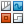to open the Library Browser. In the Library Browser, select the System Identification Toolbox library. The right side of the window displays blocks specific to the System Identification Toolbox product.

Tip

Alternatively, to access the System Identification block library, type `slident` in the MATLAB Command Window.

4. Drag the following System Identification Toolbox blocks to the new model window:

• IDDATA Sink block

• IDDATA Source block

• IDMODEL model block

5. In the Simulink Library Browser, select the Simulink > Sinks library, and drag the Scope block to the new model window.

6. In the Simulink model window, connect the blocks to resembles the following figure.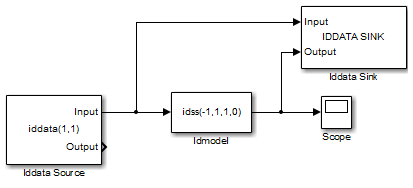Next, you configure these blocks to get data from the MATLAB workspace and set the simulation time interval and duration.

#### Configuring Blocks and Simulation Parameters

This procedure guides you through the following tasks to configure the model blocks:

• Getting data from the MATLAB workspace.

• Setting the simulation time interval and duration.

1. In the Simulink Editor, select Modeling > Model Settings > Model Settings Ctrl+E.

2. In the Configuration Parameters dialog box, in the Solver subpane, in the Stop time field, type `200`. Click .

This value sets the duration of the simulation to 200 seconds.

3. Double-click the Iddata Source block to open the Source Block Parameters: Iddata Source dialog box. Then, type the following variable name in the IDDATA object field:

`z_input`

This variable is the data object in the MATLAB workspace that contains the input data.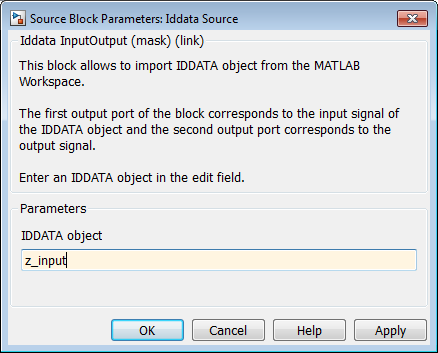Tip

As a shortcut, you can drag and drop the variable name from the MATLAB Workspace browser to the IDDATA object field.

Click .

4. Double-click the Idmodel block to open the Function Block Parameters: Idmodel dialog box.

1. Type the following variable name in the Model variable field:

`P2DUe2`

This variable represents the name of the model in the MATLAB workspace.

2. Clear the Add noise check box to exclude noise from the simulation. Click .

When Add noise is selected, Simulink derives the noise amplitude from the `NoiseVariance` property of the model and adds noise to the model accordingly. The simulation propagates this noise according to the noise model H that was estimated with the system dynamics:

`$H=\frac{C}{D}e$`Click .

5. Double-click the Iddata Sink block to open the Sink Block Parameters: Iddata Sink dialog box. Type the following variable name in the IDDATA Name field:

`z_sim_out`

6. Type `1` in the Sample Time (sec.) field to set the sample time of the output data to match the sample time of the input data.Click .

The resulting change to the Simulink model is shown in the following figure.#### Running the Simulation

1. In the Simulink Editor, select Simulation > Run.

2. Double-click the Scope block to display the time plot of the model output.3. In the MATLAB Workspace browser, notice the variable `z_sim_out` that stores the model output as an `iddata` object. You specified this variable name when you configured the Iddata Sink block.

This variable stores the simulated output of the model, and it is now available for further processing and exploration.